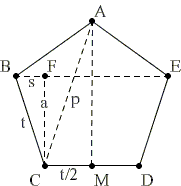# Pentagon Proportions Sangaku

Due to the design of my logo, this simple sangaku is quite dear to my heart. (The problem was described and solved in volume 3 of a 1810 text by Tenshoho Sinan and Anmei Aida, Geometry and Algebra, see [Fukagawa & Pedoe, Example 4.3].)Construct a regular pentagon by tying a knot in a strip of paper of width a. Calculate the side t of the pentagon in terms of a.

The method of construction is "right over left and under". The paper is then smoothed out, and the pentagon appears. Billet doux (a love letter or note) were sent thus in Japan.

Solution

### References

1. H. Fukagawa, D. Pedoe, Japanese Temple Geometry Problems, The Charles Babbage Research Center, Winnipeg, 1989

Write to:

Charles Babbage Research Center
P.O. Box 272, St. Norbert Postal Station
Winnipeg, MB## SangakuConstruct a regular pentagon by tying a knot in a strip of paper of width a. Calculate the side t of the pentagon in terms of a.

### Solution

Label the points and lengths as in the diagram below:Since ∠BCF = ∠CAM

BF : BC = CM : AC,

or

s : t = t/2 : p.

But p = t + 2s, from which

t = (1 + 5) s.

(Since ∠BCF = 18°, this just says that sin(18°) = (5 - 1) / 4, as we know.)

From BC² = BF² + FC², we successively get

t² = [t / (1 + 5)]² + a²,

a² = [(5 + 5) / 8] · t²,

a = [10 + 2√5 / 4] · t,

t = 2 - 2√(4/5) · a.

Note: after the above derivation Fukagawa and Pedoe discus an elegant construction of a regular pentagon with straight edge and compass.